www.microwavejournal.com/articles/29910-time-domain-channel-compensation-suitable-for-wideband-digital-predistortion

# Time Domain Channel Compensation Suitable for Wideband Digital Predistortion

March 14, 2018

In-phase/quadrature-phase (I/Q) errors in the implementation of a digital predistortion (DPD) system based on a direct-conversion structure have proven to be a limiting performance factor in modern communication systems. This work presents a method of time domain compensation (TDC) for frequency-dependent channel imbalances in wideband DPD systems. Compared with the widely used frequency domain compensation method, this method has lower computational complexity. Experimental results show that this method improves transceiver loop normalized mean squared error (NMSE) for 20 and 60 MHz Long Term Evolution (LTE) signals by 10.9 and 11.3 dB, respectively. Adjacent channel power ratio (ACPR) is improved by more than 6 and 8 dB, respectively.

The RF power amplifier (PA) is a major component in wireless communication systems; however, PA intermodulation distortion leads to signal errors and spectrum expansion. To solve the problem, both analog and digital predistortion have been employed.1,2 DPD, with the advantages of convenient implementation, flexibility and adaptability, has become the preferred approach.Figure 1 DPD system with an imperfect transceiver loop.

Figure 1 is a block diagram of a DPD system with an imperfect transceiver loop. Numerous DPD models have been presented,3 but they do not consider the impact of I/Q imbalance in the transceiver. Anttila et al.4 noted that I/Q imbalance seriously affects DPD performance, and they presented a new DPD structure for reducing I/Q imbalance in direct-conversion radio transmitters. Kim et al.5 described a joint DPD method for compensating PA nonlinearity and I/Q imbalance; however, only frequency-independent I/Q imbalance was considered. Rampa6 introduced a frequency-dependent I/Q imbalance compensation scheme, but considered only transmitter distortion. Rawat et al.7 used a hybrid RF/DPD structure based on a lookup table to compensate for I/Q imbalance, but it needed a large amount of storage space.

In this work, we focus on PA nonlinearity and frequency-dependent I/Q imbalance in the implementation of a broadband DPD platform that compensates for imbalance from both the transmitter and receiver. Through measurements of the signal with different bandwidths, the method of TDC described here works well in systems with significant I/Q distortion.NONLINEAR DISTORTION MODEL

Figure 2 shows the block diagram of a typical direct-conversion receiver to illustrate the following discussion of I/Q receiving channel imbalance model extraction and PA nonlinear modeling.

Channel I/Q Imbalance Model

Without loss of generality, the following derivation assumes that the I/Q imbalance is frequency independent. Also assume that the I/Q imbalance is caused by local oscillator (LO) imbalance in the I/Q path. Figure 2 shows the baseband equivalent signal model for I/Q imbalance, which can be written as1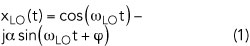Using Euler’s formulaand α is the amplitude imbalance and φ is the phase imbalance.

Actually, Equation 2 takes into account the bandpass filter (BPF) imbalance as well. Assuming that the received signal is ideal, where xI(n) and xQ(n) are the real and imaginary parts, the complex baseband signal isIn the receiver, the ideal complex baseband signal through the BPF and mixer can be written asand we havefor which the matrix form isIf α and φ are extracted from Equation 1, the amount of compensation for the channel can be estimated. In most of the literature, compensation is done with a mirror image signal. Alternatively, we use digital pre-compensation in the I and Q paths.

PA Model Extraction

The nonlinear model of a PA is also key to DPD performance. However, because much has been written about this in the literature,8-10 it is not the focus of our work. We use the first-order dynamic derivation reduction (DDR) model to describe PA nonlinearity, which can be written as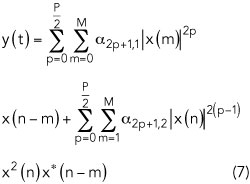where p denotes the order of nonlinearity and M is the memory length. x(n) and y(n) are the input and output of the PA, respectively. From Equation 7, the matrix form is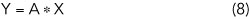where X is the PA input vector and Y is the PA output vector. A represents the coefficients vector of the PA, which can be obtained with the least squares algorithm. For example,CHANNEL COMPENSATION MODELING

Frequency-dependent I/Q imbalance is modeled to compensate for an imperfect channel. I/Q imbalance compensation modeling is separated for the receiver and transmitter channels.

In the receiver, TDC amplitude and phase for the wideband channel are extracted by constructing an orthogonal and cyclically symmetric signal. Wideband time domain modeling is performed by using compensation values for different frequency bands. The real and imaginary parts of the test signal satisfywhere E{} is the mathematical expectation signal. After obtaining α and φ, we solve the inverse matrix of Equation 13 to obtain a frequency-independent TDC expression for the receiving channel.Figure 3 Wideband compensation model for the receiver.

The wideband compensation model (see Figure 3) can be regarded as a combination of the corresponding compensation coefficients for each frequency point. From Figure 3, the expression for compensation can be written aswhere hRIf(z) and hRQf(z) are the I/Q channel compensation functions. They are implemented using a finite impulse response (FIR) structure. In Figure 3, the z-N/2 block eliminates the delay of the I and Q paths, and N represents the FIR order.

Transmitting Channel Compensation Model

Because the imbalance of the transmission channel is caused mainly by the physical circuit, external interference is very small. Therefore, the imbalance of the transmit channel is much flatter than that of the receiving channel. In order to compensate for a wideband transmit signal, we use a derivation based on polar coordinates (see Figure 4).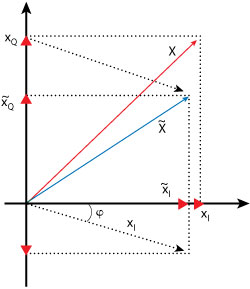Figure 4 Polar coordinate model for transmitter channel compensation.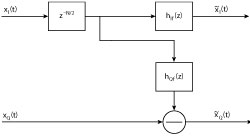Figure 5 Wideband compensation model for the transmitter.

Assume the I path offset angle is  φ. From Figure 5, the relationship of and x̃I and  x̃Q (compensated output) with the ideal input signal isExtended to a frequency-dependent compensation expression,where hSIf(z) and hSQf(z) are the I/Q channel compensation functions. They are implemented using a FIR structure. The z-N/2 block eliminates the delay of I and Q paths, where N represents the order of FIR. Compensation is performed in the baseband I and Q paths.

VALIDATION AND EXPERIMENTAL RESULTS

First, the compensation effect of the transceiver channel was verified, followed by verification of the DPD effect on the signal before and after transceiver channel compensation. Figure 6 shows the software and hardware architecture of the DPD platform. Validation used 20 MHz single-carrier and 60 MHz three-carrier LTE signals with peak-to-average power ratios (PAPR) of 7.25 and 9.29 dB, respectively. All original signals generated in MATLAB were fed to the PA through an FPGA.Figure 6 Software and hardware architecture of the DPD platform.

A PA designed with a Wolfspeed (Cree) CGH40010 transistor was used for the device under test. The PA was biased with VGS = -2.7 V and VDS = 28 V, placing the amplifier in class AB mode of operation. The PA was driven to saturation with a peak output power of 40 dBm. The operating frequency band was 1.7 to 2.6 GHz, with DPD validation at 2.4 GHz.

The DPD platform consisted of a Xilinx Virtex-7 FPGA on an ADI RF board. The digital-to-analog converters (DAC) and analog-to-digital converters (ADC) had 16- and 14-bit resolution, respectively. The maximum sample rate of the ADC was 250 MSPS, and the maximum sample rate of the DAC was 1 GSPS.

Effect of Loop Compensation

AM/AM and AM/PM are used to characterize channel memory and nonlinearity. To verify the channel compensation model, the 20 and 60 MHz signals are used to test nonlinearity and memory level with and without the channel compensation model. Measured results for the AM/AM and AM/PM characteristics of the channel are shown in Figure 7.Figure 7 Measured AM/AM and AM/PM for 20 MHz single-carrier (a) and 60 MHz three-carrier (b) LTE signals.Figure 8 Measured PA output spectrum for 20 MHz single-carrier (a) and 60 MHz three-carrier (b) LTE signals.

I/Q transceiver channel imbalance causes significant signal amplitude and phase distortion in an uncompensated channel. With increased bandwidth, imbalance and resulting distortion are greater. The compensation scheme effectively reduces this distortion. Table 1 compares NMSE performance with and without compensation, showing improvements of 10.9 and 11.3 dB, respectively, for the 20 and 60 MHz LTE signals.

DPD Measurement Results

In general, DPD performance depends on the quality of the model and the accuracy of the parameter extraction process. The TDC model described extracts the PA output signal accurately. To verify that it is suitable for wideband DPD, two sets of measurements were made.

Without loss of generality, the measurements were made using the first-order DDR model. Memory depth and nonlinear order number were consistent in the measurements to objectively demonstrate the advantages of the model. Figure 8a shows the results of PA linearization for 20 MHz single-carrier LTE signals. It can be seen that the TDC model has good adaptability to frequency-dependent I/Q imbalance, resulting in better linearization performance in terms of ACPR at the output of the PA. Figure 8b shows the results of PA linearization for 60 MHz three-carrier LTE signals. With the increased bandwidth, the I/Q channel is more sensitive to DPD performance. Table 2 shows measured ACPR performance for the two bandwidths.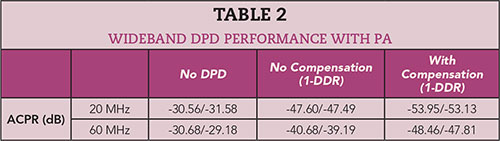CONCLUSION

Wideband channel compensation is becoming more important in communications systems. In this paper, a method of TDC for frequency-dependent channel imbalance in wideband DPD systems is described using the FIR structure in FPGA hardware. Better than 6 and 8 dB ACPR improvement was achieved with 20 and 60 MHz LTE signals, respectively, compared to performance without channel compensation.n

References

1. M. Nakayama, K. Mori, K. Yamauchi, Y. Itoh and T. Takagi, “A Novel Amplitude and Phase Linearizing Technique for Microwave Power Amplifiers,” IEEE MTT-S International Microwave Symposium Digest, May 1995, pp.1451–1454.
2. Y. Ma, S. He, Y. Akaiwa and Y. Yamao, “An Open-Loop Digital Predistorter Based on Memory Polynomial Inverses for Linearization of RF Power Amplifier,” International Journal on RF Microwowave Computer Aided Engineering, Vol. 21, No. 5, September 2011, pp. 589–595.
3. A. Zhu, J. C. Pedro and T. J. Brazil, “Dynamic Deviation Reduction-Based Volterra Behavioral Modeling of RF Power Amplifiers,” IEEE Transactions on Microwave Theory and Techniques, Vol. 54, No. 12, December 2006, pp. 4323–4332.
4. L. Anttila, P. Händel and M. Valkama, “Joint Mitigation of Power Amplifier and I/Q Modulator Impairments in Broadband Direct-Conversion Transmitters,” IEEE Transactions on Microwave Theory and Techniques, Vol. 58, No. 4, April 2010, pp. 730–739.
5. Y. D. Kim, E. R. Jeong and Y. H. Lee, “Adaptive Compensation for Power Amplifier Nonlinearity in the Presence of Quadrature Modulation/Demodulation Errors,” IEEE Transactions on Signal Processing, Vol. 55, No. 9, September 2007, pp. 4717–4721.
6. V. Rampa, “I/Q Compensation of Broadband Direct-Conversion Transmitters,” IEEE Transactions on Microwave Communications, Vol. 13, No. 6, June 2014, pp. 3329–3342.
7. K. Rawat, M. Rawat and F. M. Ghannouchi, “Compensating I–Q Imperfections in Hybrid RF/Digital Predistortion With an Adapted Lookup Table Implemented in an FPGA,” IEEE Transactions on Circuits and Systems II: Express Briefs, Vol. 57, No. 5, May 2010, pp. 389–393.
8. A. Zhu, J. Dooley and T. J. Brazil, “Simplified Volterra Series Based Behavioral Modeling of RF Power Amplifiers Using Deviation Reduction,” IEEE MTT-S International Microwave Symposium Digest, June 2006, pp. 1113–1116.
9. C. Crespo-Cadenas, J. Reina-Tosina and M. J. Madero-Ayora, “Volterra Behavioral Model for Wideband RF Amplifiers,” IEEE Transactions on Microwave Theory and Techniques, Vol. 55, No. 3, March 2007, pp. 449–457.
10. G. Xu and T. Liu, “Generalized Two-Box Cascaded Nonlinear Behavioral Model for Radio Frequency Power Amplifiers With Strong Memory Effects,” IEEE Transactions on Microwave Theory and Techniques, Vol. 62, No. 12, March 2007, pp. 449–457.